一、什么是排序算法

1.3、算法总结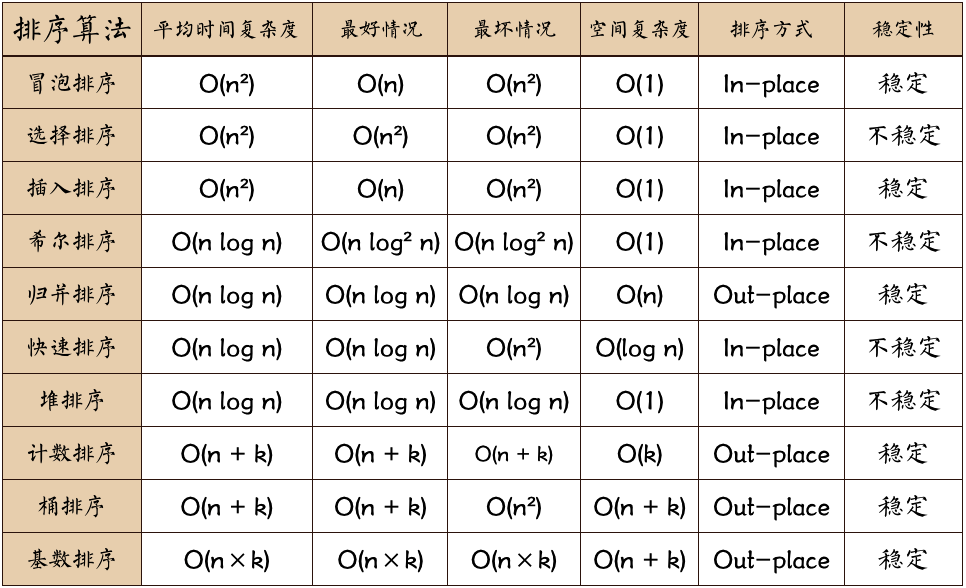（注意：n指数据规模；k指“桶”的个数；In-place指占用常数内存，不占用额外内存；Out-place指占用额外内存

1.4、算法分类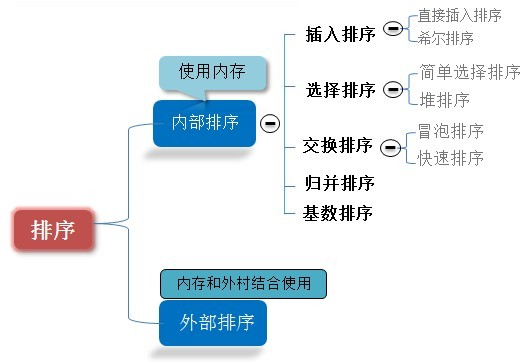二、冒泡排序（Bubble Sort）

2.1、算法描述

• 比较相邻的元素。如果第一个比第二个大，就交换它们两个；
• 对每一对相邻元素作同样的工作，从开始第一对到结尾的最后一对，这样在最后的元素应该会是最大的数；
• 针对所有的元素重复以上的步骤，除了最后一个；
• 重复步骤1~3，直到排序完成。

2.2、动图演示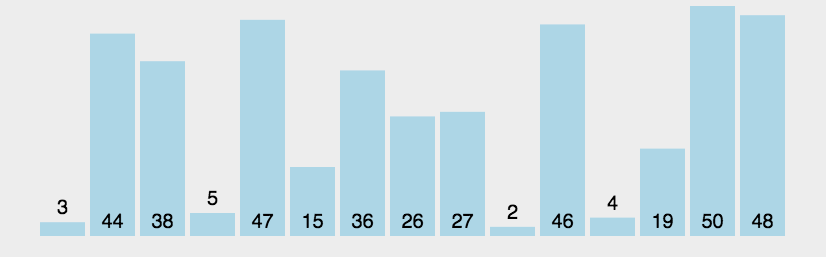2.3、代码实现

/**
* 冒泡排序
*
* @param array
* @return
*/
public static int[] bubbleSort(int[] array) {
if (array.length == 0)
return array;
for (int i = 0; i < array.length; i++)
for (int j = 0; j < array.length - 1 - i; j++)
if (array[j + 1] < array[j]) {
int temp = array[j + 1];
array[j + 1] = array[j];
array[j] = temp;
}
return array;
}

三、选择排序（Selection Sort）

3.1、算法描述

n个记录的直接选择排序可经过n-1趟直接选择排序得到有序结果。具体算法描述如下：

• 初始状态：无序区为R[1..n]，有序区为空；
• 第i趟排序(i=1,2,3…n-1)开始时，当前有序区和无序区分别为R[1..i-1]和R(i..n）。该趟排序从当前无序区中-选出关键字最小的记录 R[k]，将它与无序区的第1个记录R交换，使R[1..i]和R[i+1..n)分别变为记录个数增加1个的新有序区和记录个数减少1个的新无序区；
• n-1趟结束，数组有序化了。

3.2、动图演示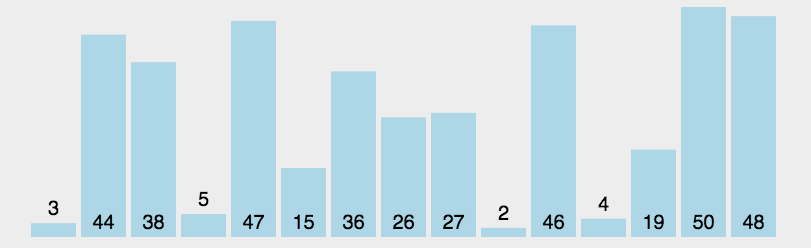3.3、代码实现

/**
* 选择排序
* @param array
* @return
*/
public static int[] selectionSort(int[] array) {
if (array.length == 0)
return array;
for (int i = 0; i < array.length; i++) {
int minIndex = i;
for (int j = i; j < array.length; j++) {
if (array[j] < array[minIndex]) //找到最小的数
minIndex = j; //将最小数的索引保存
}
int temp = array[minIndex];
array[minIndex] = array[i];
array[i] = temp;
}
return array;
}

四、插入排序（Insertion Sort）

4.1、算法描述

• 从第一个元素开始，该元素可以认为已经被排序；
• 取出下一个元素，在已经排序的元素序列中从后向前扫描；
• 如果该元素（已排序）大于新元素，将该元素移到下一位置；
• 重复步骤3，直到找到已排序的元素小于或者等于新元素的位置；
• 将新元素插入到该位置后；
• 重复步骤2~5。

4.2、动图演示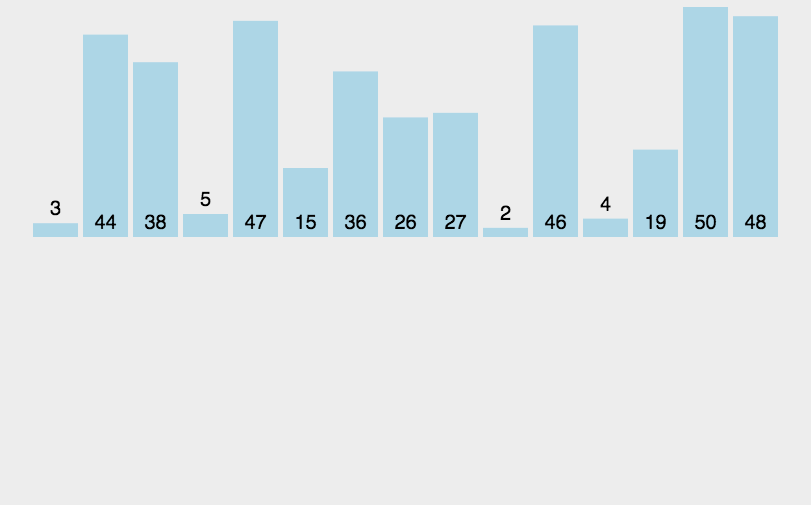4.3、代码实现

/**
* 插入排序
* @param array
* @return
*/
public static int[] insertionSort(int[] array) {
if (array.length == 0)
return array;
int current;
for (int i = 0; i < array.length - 1; i++) {
current = array[i + 1];
int preIndex = i;
while (preIndex >= 0 && current < array[preIndex]) {
array[preIndex + 1] = array[preIndex];
preIndex--;
}
array[preIndex + 1] = current;
}
return array;
}

五、希尔排序（Shell Sort）

5.1、算法描述

• 选择一个增量序列t1，t2，…，tk，其中ti>tj，tk=1；
• 按增量序列个数k，对序列进行k 趟排序；
• 每趟排序，根据对应的增量ti，将待排序列分割成若干长度为m 的子序列，分别对各子表进行直接插入排序。仅增量因子为1 时，整个序列作为一个表来处理，表长度即为整个序列的长度。

5.2、过程演示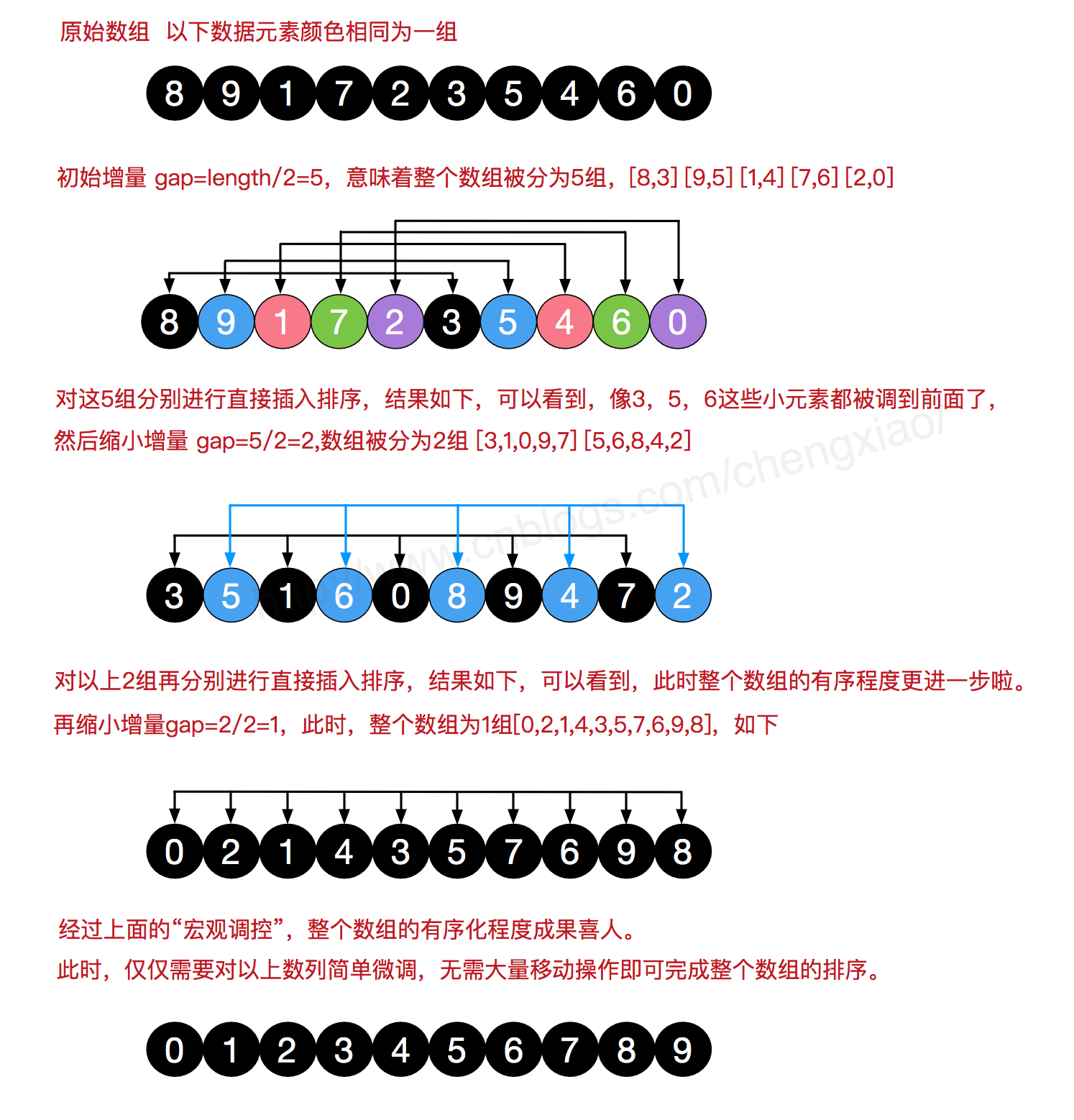5.3、代码实现

/**
* 希尔排序
*
* @param array
* @return
*/
public static int[] ShellSort(int[] array) {
int len = array.length;
int temp, gap = len / 2;
while (gap > 0) {
for (int i = gap; i < len; i++) {
temp = array[i];
int preIndex = i - gap;
while (preIndex >= 0 && array[preIndex] > temp) {
array[preIndex + gap] = array[preIndex];
preIndex -= gap;
}
array[preIndex + gap] = temp;
}
gap /= 2;
}
return array;
}

六、归并排序（Merge Sort）

6.1、算法描述

• 把长度为n的输入序列分成两个长度为n/2的子序列；
• 对这两个子序列分别采用归并排序；
• 将两个排序好的子序列合并成一个最终的排序序列。

6.2、动图演示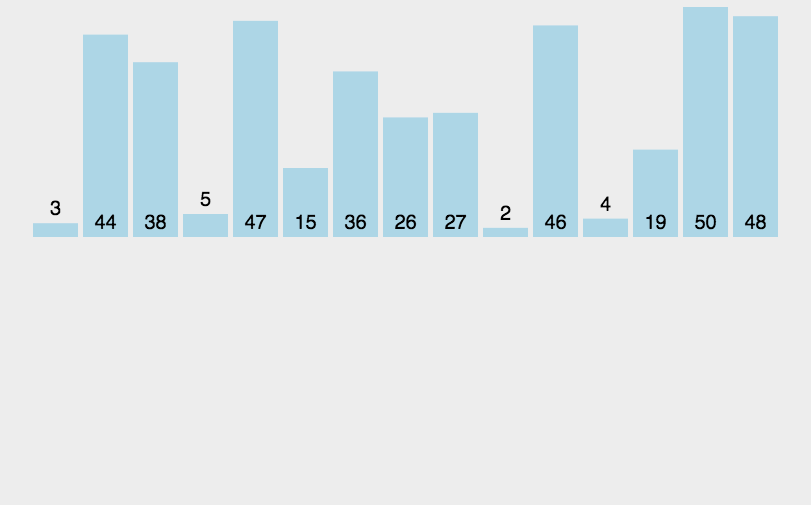6.3、代码实现

/**
* 归并排序
*
* @param array
* @return
*/
public static int[] MergeSort(int[] array) {
if (array.length < 2) return array;
int mid = array.length / 2;
int[] left = Arrays.copyOfRange(array, 0, mid);
int[] right = Arrays.copyOfRange(array, mid, array.length);
return merge(MergeSort(left), MergeSort(right));
}
/**
* 归并排序——将两段排序好的数组结合成一个排序数组
*
* @param left
* @param right
* @return
*/
public static int[] merge(int[] left, int[] right) {
int[] result = new int[left.length + right.length];
for (int index = 0, i = 0, j = 0; index < result.length; index++) {
if (i >= left.length)
result[index] = right[j++];
else if (j >= right.length)
result[index] = left[i++];
else if (left[i] > right[j])
result[index] = right[j++];
else
result[index] = left[i++];
}
return result;
}

七、快速排序（Quick Sort）

7.1、算法描述

• 从数列中挑出一个元素，称为 “基准”（pivot）；
• 重新排序数列，所有元素比基准值小的摆放在基准前面，所有元素比基准值大的摆在基准的后面（相同的数可以到任一边）。在这个分区退出之后，该基准就处于数列的中间位置。这个称为分区（partition）操作；
• 递归地（recursive）把小于基准值元素的子数列和大于基准值元素的子数列排序。

7.2、动图演示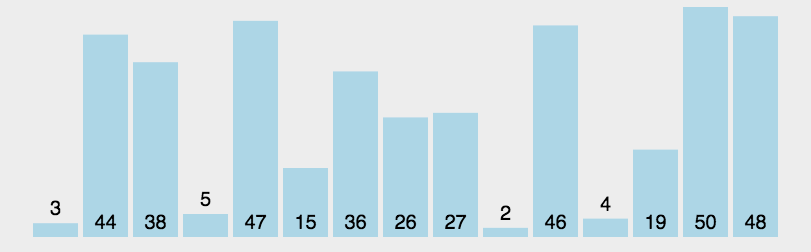7.3、代码实现

/**
* 快速排序方法
* @param array
* @param start
* @param end
* @return
*/
public static int[] QuickSort(int[] array, int start, int end) {
if (array.length < 1 || start < 0 || end >= array.length || start > end) return null;
int smallIndex = partition(array, start, end);
if (smallIndex > start)
QuickSort(array, start, smallIndex - 1);
if (smallIndex < end)
QuickSort(array, smallIndex + 1, end);
return array;
}
/**
* 快速排序算法——partition
* @param array
* @param start
* @param end
* @return
*/
public static int partition(int[] array, int start, int end) {
int pivot = (int) (start + Math.random() * (end - start + 1));
int smallIndex = start - 1;
swap(array, pivot, end);
for (int i = start; i <= end; i++)
if (array[i] <= array[end]) {
smallIndex++;
if (i > smallIndex)
swap(array, i, smallIndex);
}
return smallIndex;
}

/**
* 交换数组内两个元素
* @param array
* @param i
* @param j
*/
public static void swap(int[] array, int i, int j) {
int temp = array[i];
array[i] = array[j];
array[j] = temp;
}

8.1、算法描述

• 将初始待排序关键字序列(R1,R2….Rn)构建成大顶堆，此堆为初始的无序区；
• 将堆顶元素R与最后一个元素R[n]交换，此时得到新的无序区(R1,R2,……Rn-1)和新的有序区(Rn),且满足R[1,2…n-1]<=R[n]；
• 由于交换后新的堆顶R可能违反堆的性质，因此需要对当前无序区(R1,R2,……Rn-1)调整为新堆，然后再次将R与无序区最后一个元素交换，得到新的无序区(R1,R2….Rn-2)和新的有序区(Rn-1,Rn)。不断重复此过程直到有序区的元素个数为n-1，则整个排序过程完成。

8.2、动图演示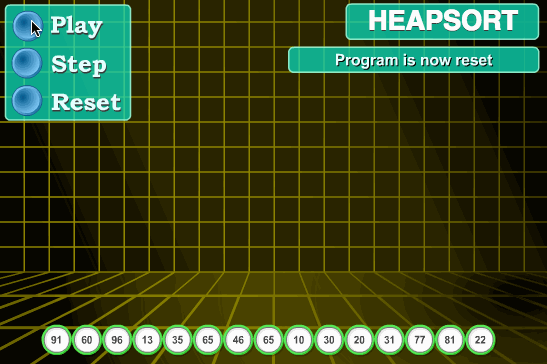8.3、代码实现

//声明全局变量，用于记录数组array的长度；
static int len;
/**
* 堆排序算法
*
* @param array
* @return
*/
public static int[] HeapSort(int[] array) {
len = array.length;
if (len < 1) return array;
//1.构建一个最大堆
buildMaxHeap(array);
//2.循环将堆首位（最大值）与末位交换，然后在重新调整最大堆
while (len > 0) {
swap(array, 0, len - 1);
len--;
}
return array;
}
/**
* 建立最大堆
*
* @param array
*/
public static void buildMaxHeap(int[] array) {
//从最后一个非叶子节点开始向上构造最大堆
for (int i = (len/2 - 1); i >= 0; i--) { //感谢 @让我发会呆 网友的提醒，此处应该为 i = (len/2 - 1)
}
}
/**
* 调整使之成为最大堆
*
* @param array
* @param i
*/
public static void adjustHeap(int[] array, int i) {
int maxIndex = i;
//如果有左子树，且左子树大于父节点，则将最大指针指向左子树
if (i * 2 < len && array[i * 2] > array[maxIndex])
maxIndex = i * 2;
//如果有右子树，且右子树大于父节点，则将最大指针指向右子树
if (i * 2 + 1 < len && array[i * 2 + 1] > array[maxIndex])
maxIndex = i * 2 + 1;
//如果父节点不是最大值，则将父节点与最大值交换，并且递归调整与父节点交换的位置。
if (maxIndex != i) {
swap(array, maxIndex, i);
}
}

8.4、算法分析

9.1、算法描述

• 找出待排序的数组中最大和最小的元素；
• 统计数组中每个值为i的元素出现的次数，存入数组C的第i项；
• 对所有的计数累加（从C中的第一个元素开始，每一项和前一项相加）；
• 反向填充目标数组：将每个元素i放在新数组的第C(i)项，每放一个元素就将C(i)减去1。

9.2、动图演示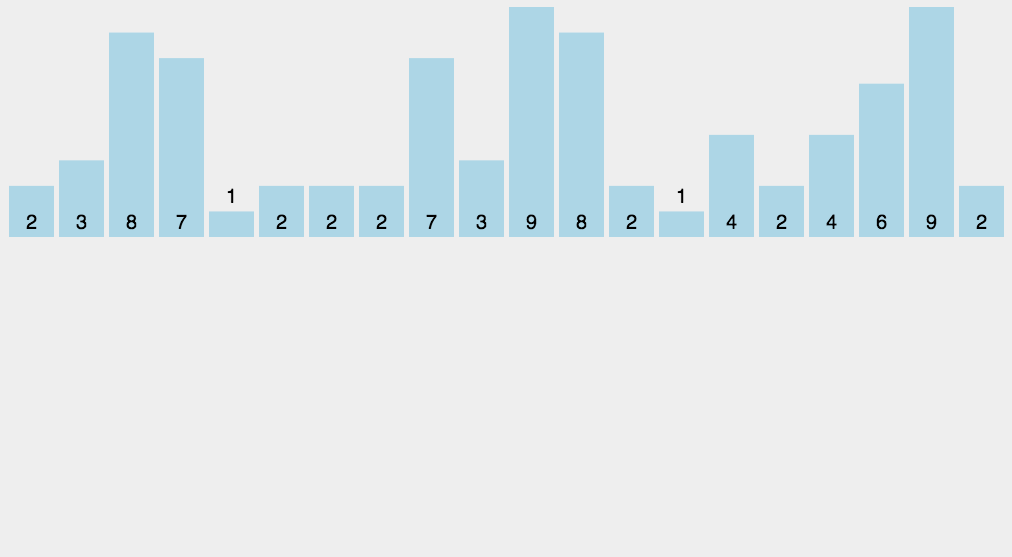9.3、代码实现

/**
* 计数排序
*
* @param array
* @return
*/
public static int[] CountingSort(int[] array) {
if (array.length == 0) return array;
int bias, min = array, max = array;
for (int i = 1; i < array.length; i++) {
if (array[i] > max)
max = array[i];
if (array[i] < min)
min = array[i];
}
bias = 0 - min;
int[] bucket = new int[max - min + 1];
Arrays.fill(bucket, 0);
for (int i = 0; i < array.length; i++) {
bucket[array[i] + bias]++;
}
int index = 0, i = 0;
while (index < array.length) {
if (bucket[i] != 0) {
array[index] = i - bias;
bucket[i]--;
index++;
} else
i++;
}
return array;
}

10.1、算法描述

• 人为设置一个BucketSize，作为每个桶所能放置多少个不同数值（例如当BucketSize==5时，该桶可以存放｛1,2,3,4,5｝这几种数字，但是容量不限，即可以存放100个3）；
• 遍历输入数据，并且把数据一个一个放到对应的桶里去；
• 对每个不是空的桶进行排序，可以使用其它排序方法，也可以递归使用桶排序；
• 从不是空的桶里把排好序的数据拼接起来。

10.2、图片演示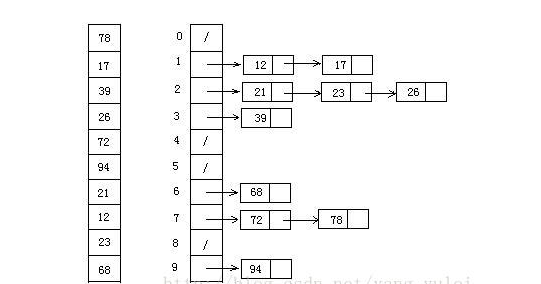10.3、代码实现

/**
* 桶排序
*
* @param array
* @param bucketSize
* @return
*/
public static ArrayList<Integer> BucketSort(ArrayList<Integer> array, int bucketSize) {
if (array == null || array.size() < 2)
return array;
int max = array.get(0), min = array.get(0);
// 找到最大值最小值
for (int i = 0; i < array.size(); i++) {
if (array.get(i) > max)
max = array.get(i);
if (array.get(i) < min)
min = array.get(i);
}
int bucketCount = (max - min) / bucketSize + 1;
ArrayList<ArrayList<Integer>> bucketArr = new ArrayList<>(bucketCount);
ArrayList<Integer> resultArr = new ArrayList<>();
for (int i = 0; i < bucketCount; i++) {
}
for (int i = 0; i < array.size(); i++) {
bucketArr.get((array.get(i) - min) / bucketSize).add(array.get(i));
}
for (int i = 0; i < bucketCount; i++) {
if (bucketSize == 1) { // 如果带排序数组中有重复数字时  感谢 @见风任然是风 朋友指出错误
for (int j = 0; j < bucketArr.get(i).size(); j++)
} else {
if (bucketCount == 1)
bucketSize--;
ArrayList<Integer> temp = BucketSort(bucketArr.get(i), bucketSize);
for (int j = 0; j < temp.size(); j++)
}
}
return resultArr;
}

11.1、算法描述

• 取得数组中的最大数，并取得位数；

11.2、动图演示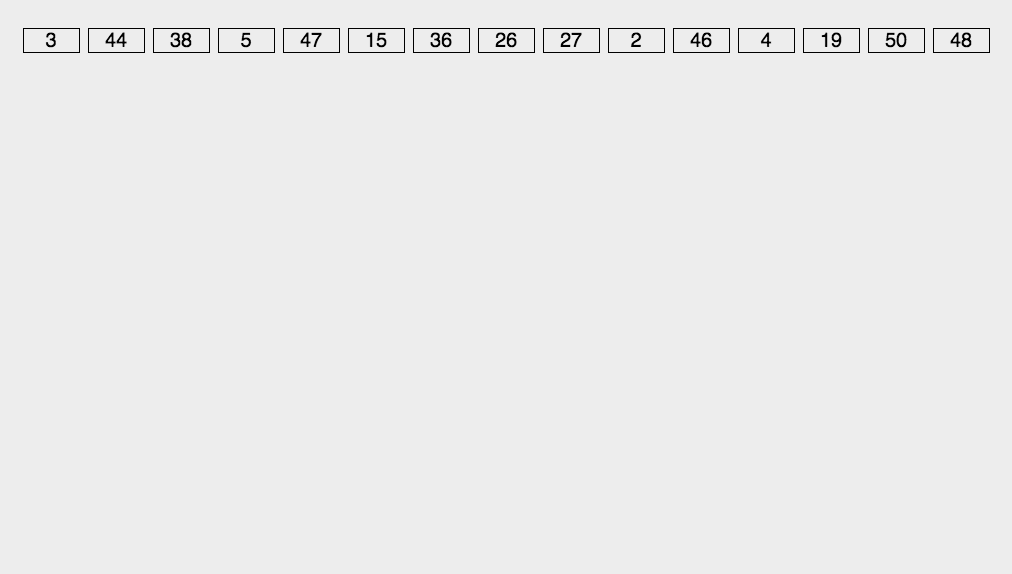11.3、代码实现

/**
* 基数排序
* @param array
* @return
*/
public static int[] RadixSort(int[] array) {
if (array == null || array.length < 2)
return array;
// 1.先算出最大数的位数；
int max = array;
for (int i = 1; i < array.length; i++) {
max = Math.max(max, array[i]);
}
int maxDigit = 0;
while (max != 0) {
max /= 10;
maxDigit++;
}
int mod = 10, div = 1;
ArrayList<ArrayList<Integer>> bucketList = new ArrayList<ArrayList<Integer>>();
for (int i = 0; i < 10; i++)
for (int i = 0; i < maxDigit; i++, mod *= 10, div *= 10) {
for (int j = 0; j < array.length; j++) {
int num = (array[j] % mod) / div;
}
int index = 0;
for (int j = 0; j < bucketList.size(); j++) {
for (int k = 0; k < bucketList.get(j).size(); k++)
array[index++] = bucketList.get(j).get(k);
bucketList.get(j).clear();
}
}
return array;
}

11.4、算法分析

• 基数排序：根据键值的每位数字来分配桶
• 计数排序：每个桶只存储单一键值
• 桶排序：每个桶存储一定范围的数值

0 个人打赏©️2019 CSDN 皮肤主题: 技术黑板 设计师: CSDN官方博客